# Chaos in Excel

I found a book about chaos (being the branch of mathematics, as opposed to the US government's response to the hurricane) in the local library, and knowing nothing about it (other than having seen pretty fractal pictures) decided to check it out.

In the book, there was a formula for predicting a theoretical population change, where the next year's population equals `rx(1-x)`, where x is between zero and one. Zero is extinction, and one is the absolute maximum the population could be. I can't remember what r means, and I can't get the book without waking Julia.

Anyway, I thought I'd try this out in Excel, as it looked easy. If you want to join in at home, here's what to do.

1. In a new spreadsheet, put the value 2.7 in cell A1 (r).
2. Put the value 0.02 in cell B1 (initial x)
3. In B2, put the formula `=\$A\$1*B1*(1-B1)`
4. Select cell B2, and drag the small square in its bottom right corner down a few hundred rows (or right to the bottom if you can be bothered)
5. Insert > Chart > Line > [first type] > Next
6. Set the data range to be `\$B\$1:\$B\$500`
7. Next > Next > As object in > Finish

If my instructions are correct, you should have a graph that starts low, leaps high, and zig-zags a few times before settling at about 0.629.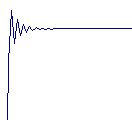Changing A1 to 3.0 produced a graph that zig-zagged between two values that appeared to be converging very slowly. So slowly, that at row 65536 they still hadn't met.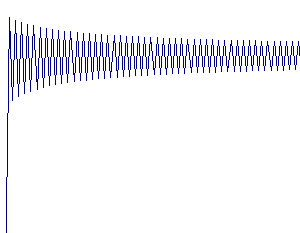At 3.5, the zig-zags take on four values, rather than two (I've change the scaling for clarity):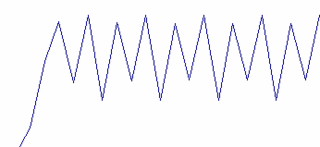At 3.57, there seem to be eight values: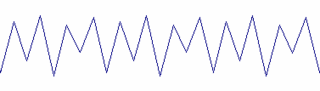At 3.61, I can't really work out how many values there are, or how often they occur, but the graph looks like a combination of the previous ones. Looking at the rows at the bottom, the spacing of numbers starting with 0.7 are 26, 14, 10, 28, 20, 18, 10, 10, 12, which seems random to me.At 3.71, new shapes are starting to appear in the chart: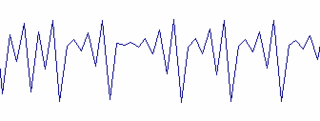At 4.0, a curved shape appears: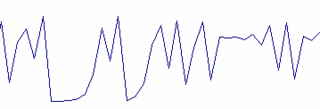Now for the chaotic bit. There's a bit more stuff to do in Excel here:

1. In C1, put the formula `=B1`
2. In A2, put the formula `=A1+0.000000001`
3. In C2, put the formula `=(\$A\$2)*C1*(1-C1)`
4. Double click the small black square in the bottom right corner of C2 (which should fill down the formula)
5. Set the data range of the chart to be \$B\$1:\$C\$500

What we've done here is to draw a new line on the graph, which compares 4 with 4.000000001. You'd think they'd be pretty similar, and they are to start with, but the small difference gets multiplied up really quickly, and the lines soon go their separate ways. After only 37 points, the values are more than 0.9 apart, within a range of 0.0 to 1.0!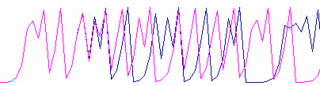The lines become completely unrelated to each other, and this is the essence of chaos. A change of one billionth can affect the line of a really simple formula in so few values. This is the numerical equivalent of the butterfly flapping its wings in
Doddiscombsleigh affecting George Bush's opinion polls ratings.

Anyway, if you've got this far, have a play about with different values of r and x, and see what happens.

I'm not a mathematician, so this is all brand new to me, and really rather intriguing. I don't understand why this does what it does, but I'm going to be mulling it over for a while yet.

11 September 2005